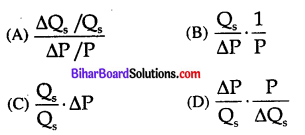# Bihar Board 12th Economics Objective Answers Chapter 3 Producer Behaviour and Supply

Bihar Board 12th Economics Objective Questions and Answers

## Bihar Board 12th Economics Objective Answers Chapter 3 Producer Behaviour and Supply

Question 1.
In production function, production is a function of:
(A) Price
(B) Factors of Production
(C) Total Expenditure
(D) None of these
(B) Factors of ProductionQuestion 2.
The basic reason of operating the Law of Diminishing Returns is:
(A) Scarcity of Factors
(B) Imperfect Substitution between Factors
(C) Both (a) and (b)
(D) None of the above
(C) Both (a) and (b)

Question 3.
Which of the following explains the short-run production function ?
(A) Law of Demand
(B) Law of Variable Proportion
(C) Returns to Scale
(D) Elasticity of Demand
(B) Law of Variable ProportionQuestion 4.
Long-run production function is related to:
(A) Law of Demand
(B) Law of Increasing Returns
(C) Laws of Returns to Scale
(D) Elasticity of Demand
(C) Laws of Returns to Scale

Question 5.
In which stage of production a rational producer likes to operate in shot-run production ?
(A) First Stage
(B) Second Stage
(C) Third Stage
(D) None of these
(B) Second Stage

Question 6.
Law of variable proportion explains three stages of production. In the first stage of production:
(A) Both MP and AP rise
(B) MP rises
(C) AP Falls
(D) MP is zero
(A) Both MP and AP riseQuestion 7.
At which time all the factors of production may be changed ?
(A) Short run
(B) Long run
(C) Very Long run
(D) All the three
(B) Long run

Question 8.
Production function is expressed as:
(A) Qx = Px
(B) Qx = f(A, B, C, D)
(C) Qx = Dx
(D) None of these
(B) Qx = f(A, B, C, D)

Question 9.
Which factors among following we find in short-run production process ?
(A) Fixed Factors
(B) Variable Factors
(C) Both (a) and (b)
(D) None of these
(C) Both (a) and (b)

Question 10.
The cycle which increases first and after being constant starts to reduce is called :
(A) APP
(B) MPP
(C) TPP
(D) All these
(D) All theseQuestion 11.
Which of the following is a saurce of production ?
(A) Land
(B) Labour
(C) Capital
(D) All of these
(D) All of these

Question 12.
Law of variable proportion is related to :
(A) Both short-run and long run
(B) Long-run
(C) Short-run
(D) Very Long-run
(C) Short-run

Question 13.
An active factor of production is:
(A) Capital
(B) Labour
(C) Land
(D) None of these
(B) Labour

Question 14.
If all the factors of production are increased by same proportion and as a result output increases by a greater proportion than it is called :
(A) Constant returns to scale
(B) Decreasing returns to scale
(D) All of these
(D) None of these
(D) All of these

Question 15.
Which of the following is included in money cost ?
(A) Normal Profit
(B) Explicit Cost
(C) Implicit Cost
(D) All of these
(D) All of theseQuestion 16.
Which of the following is not fixed cost ?
(B) Interest
(C) Cost of Raw Material
(D) Rent of the Factory
(C) Cost of Raw Material

Question 17.
With the increase in production the difference between total cost and total fixed cost:
(A) Remains Constant
(B) Increases
(C) Decreases
(D) Both Increases or Decreases
(B) Increases

Question 18.
Changes in production quantity affect:
(A) Both Fixed and Variable Cost
(B) Only Variable Cost
(C) Only Fixed Cost
(D) None of the above
(B) Only Variable Cost

Question 19.
What happens when production is shut down ?
(A) Fixed Cost Increases
(B) Variable Costs Decline
(C) Variable Costs become zero
(D) Fixed Costs become zero
(C) Variable Costs become zeroQuestion 20.
The alternative name of opportunity cost is:
(A) Economic Cost
(B) Equilibrium Price
(C) Marginal Cost
(D) Average Cost
(A) Economic Cost

Question 21.
When average cost is decreasing what status marginal cost has as compared to average cost ?
(A) MC > AC
(B) MC = AC
(C) MC ≤ AC
(D) MC ≠ AC
(C) MC ≤ AC

Question 22.
Which statement of the following is true ?
(A) AC= TFC – TVC
(B) AC = AFC + TVC
(C) AC= TFC + AVC
(D) AC = AFC + AVC
(D) AC = AFC + AVC

Question 23.
What is an opportunity cost ?
(A) The alternative foregon
(B) The opportunity lost
(C) Transfer earnings
(D) All of these
(D) All of these

Question 24.
The shape of average cost curve is :
(A) U-shaped
(B) Reactangular Hyperbola shaped
(C) Line parallel to x-axis
(D) None of these
(A) U-shapedQuestion 25.
The average fixed cost at 5 units of output is Rs. 20. Average variable cost at 5 units of output is Rs. 40. Average cost of producing 5 units is:
(A) Rs. 20
(B) Rs. 40
(C) Rs.56
(D) Rs.60
(D)Rs.60

Question 26.
Which of the following is correct ?
(A) TVC = TC – TFC
(B) TC = TVC-TFC
(C) TFC = TVC + TC
(D) TC = TVC x TFC
(A) TVC = TC – TFC

Question 27.
Average variable costs can be defined as:
(A) TVC x Q
(B) TVC + Q
(C) TVC-Q
(D) TVC ÷ Q
(D) TVC ÷ Q

Question 28.
With increase in output, the difference between total cost and total variable cost:
(A) Decreases
(B) Increases
(C) Remains Constant
(D) None of the above
(C) Remains ConstantQuestion 29.
Which factors are used in short-run production process ?
(A) Fixed Factors
(B) Variable Factors
(C) Both (a) and (b)
(D) None of the above
(C) Both (a) and (b)

Question 30.
Following figure shows: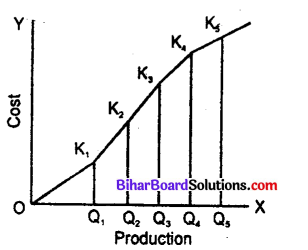(A) Total Fixed Cost
(B) Total Variable Cost
(C) Total Cost
(D) None of these
(B) Total Variable Cost

Question 31.
In which market MR may become zero or negative ?
(A) Monopoly
(B) Monopolistic Competition
(C) Both (a) and (b)
(D) Perfect Competition
(C) Both (a) and (b)

Question 32.
In which market AR = MR ?
(A) Monopoly
(B) Monopolistic Competition
(C) Both (a) and (b)
(D) Perfect Competition
(D) Perfect CompetitionQuestion 33.
In monopoly and monopolistic competition :
(A) AR = MR
(B) AR > MR
(C) AR < MR (D) None of these Answer: (B) AR > MR

Question 34.
To which market, following figure belongs ?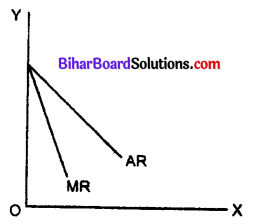(A) Perfect Competition
(B) Monopoly
(C) Monopolistic Competition
(D) None of the above
(B) Monopoly

Question 35.
With which condition, firm will get maximum profit ?
(A) Where MR = MC
(B) Where MC cuts MR from below
(C) Both (a) and (b)
(D) None of the above
(C) Both (a) and (b)

Question 36.
In perfect competition, which of the following remains constant ?
(A) AR
(B) MR
(C) Both AR and MR
(D) None of the both
(C) Both AR and MRQuestion 37.
In perfect competition:
(A) AR = MR
(B) AR > MR
(C) MR < MC
(D) MR = MC = 0
(A) MR = MC

Question 38.
When 5 units of a goods are sold, total revenue is Rs. 100. When 6 units are sold, marginal revenue is Rs. 8. At what price are 6 units sold ?
(A) Rs. 28 per unit
(B) Rs. 20 per unit
(C) Rs. 18 per unit
(D) Rs. 12 per unit
(C) Rs. 18 per unit

Question 39.
MR is shown as: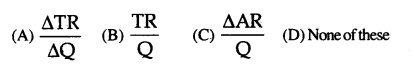(A)

Question 40.
AR is shown as:(A)

Question 41.
In which market AR curve is parallel to X-axis ?
(A) Perfect Competition
(B) Monopoly
(C) Monopolistic Competition
(D) In all the above
(A) Perfect Competition

Question 42.
Which of the following is a true statement ?
(A) AR indicates price
(B) AR Curve and Demand Curve are the same
(C) Both (a) and (b)
(D) None of the above
(C) Both (a) and (b)

Question 43.
The basic condition of firm’s equilibrium is:
(A) MC = MR
(B) MR = TR
(C) MR = AR
(D) AC = AR
(A) MC = MR

Question 44.
In final equilibrium of firm:
(A) MC cuts MR from above
(B) MC cuts MR from below
(C) Both (a) and (b) are
(D) None of the above is true
(B) MC cuts MR from below

Question 45.
For every market, which condition has to be fulfilled for firm’s equilibrium ?
(A) AR = MC
(B) MR = MC
(C) MC should cut MR from below
(D) Both (b) and (c)
(D) Both (b) and (c)

Question 46.
Which is a method of producer’s equilibrium ?
(A) TR and TC Method
(B) MR and MC Method
(C) Both (a) and (b)
(D) None of the above
(C) Both (a) and (b)

Question 47.
For a firm’s equilibrium:
(A) MR = MC
(B) MR > MC
(C) MR < MC
(D) MR = MC = 0
(A) MR = MC

Or

The first condition of firm’s equilibrium is :
(A) MC = MR
(B) MR = TR
(C) MR = AR
(D) AC = AR
(A) MC = MR

Question 48.
On which assumption, the law of supply depends ?
(A) There should be no change in income levels of buyers and sellers in the market.
(B) Prices of factors of production remain stable
(C) Technological level remains constant
(D) All the above
(D) All the above

Question 49.
If other things being same, what does the positive relationship between price and supply quantity signify ?
(A) Law of Demand
(B) Elasticity of Supply
(C) Law of Supply
(D) Supply Function
(C) Law of SupplyQuestion 50.
The reason of decrease in supply is:
(A) Increase in Production Cost
(B) Increase in Price of Substitutes
(C) Fall in number of Firms in the Industry
(D) All the above
(D) All the above

Question 51.
The figure given below shows: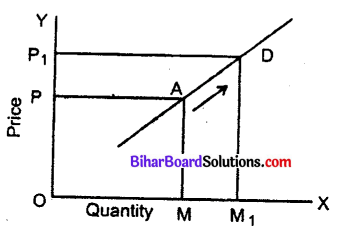(A) Extension in Supply
(B) Contraction in Supply
(C) Elasticity of supply
(D) Elasticity of demand
(A) Extension in Supply

Question 52.
The quantity of a goods which the seller is ready to sell in the market at fixed price and time is called ?
(A) Supply
(B) Demand
(C) Elasticity of supply
(D) Elasticity of Demand
(A) Supply

Question 53.
Supply is associated with:
(A) A Time Period
(B) Price
(C) Both (a) and (b)
(D) None of the above
(C) Both (a) and (b)Question 54.
Determinating factor of supply of goods is:
(A) Price of Goods
(B) Price of Related Goods
(C) Price of Factor of Production
(D) All the above
(D) All the above

Question 55.
Which of the following statement is true ?
(A) Price and quantity have direct relationship
(B) Supply curve rises from left to right
(C) Supply is affected by many factors
(D) All the above
(D) All the above

Question 56.
Which of the following function shows the laws of supply ?
(A) S = f(P)
(B) S = f(a/p)
(C) S =f(Q)
(D) None of the above
(A) S = f(P)

Question 57.
Following figure shows :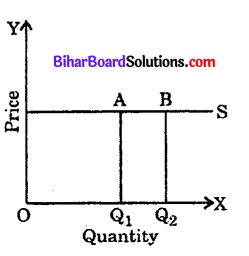(A) Perfectly Elastic Supply
(B) Perfectly Inelastic Supply
(C) Elastic Supply
(D) Inelastic Supply
(A) Perfectly Elastic SupplyQuestion 58.
Which of the following is correct ?
(A) Perfectly Elastic Supply es = ∞
(B) High Elastic Supply es > 1
(C) Perfectly Inelastic Supply es = 0
(D) All the above
(D) All the above

Question 59.
es = 0 means that elasticity of supply is:
(A) Perfectly Elastic Supply
(B) Perfectly Inelastic Supply
(C) Less Elastic Supply
(D) Unit Elastic Supply
(B) Perfectly Inelastic Supply

Question 60.
If the price of goods rises by 60% but supply increases by only 5%, the supply of goods will be:
(A) Highly Elastic
(B) Elastic
(C) Inelastic
(D) Perfectly Inelastic
(C) Inelastic

Question 61.
The elasticity of a straight line supply curve originating from the centre of origin is:
(A) Less than unity,
(B) greater than unity
(C) equal to unity
(D) equal to zero
(C) equal to unityQuestion 62.
When supply increases more with a result of small increase in price, the nature of supply will be :
(A) Elastic
(B) Inelastic
(C) Perfectly Elastic
(D) Perfectly Inelastic
(A) Elastic

Question 63.
When the proportionate change in the supply of goods is more than the proportionate change in its price, the elasticity of supply will be:
(A) Less than Unit
(B) Equal to Unit
(C) Greater than Unit
(D) Infinite
(C) Greater than Unit

Question 64.
If the price of the goods rises by 60% and supply increases by only 5%, the supply of goods will be :
(A) Highly Elastic
(B) Elastic
(C) Inelastic
(D) Perfectly Inelastic